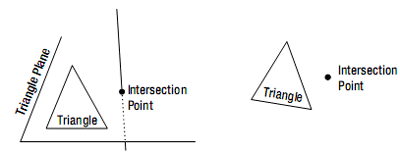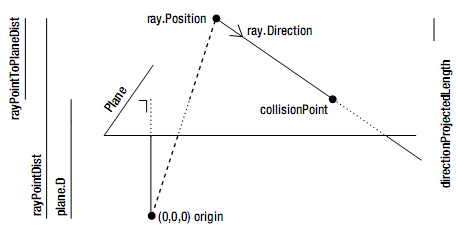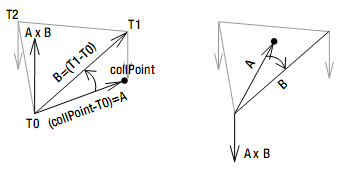# 4.18 使用逐三角形检查检测射线-模型碰撞

## 工作原理

6. 将新创建的引用添加到项目中。

7. 选择新创建的处理器处理模型。

8. 设置项目依赖项。

9. 在XNA项目中，添加内容管道的引用。

10. 添加内容管道命名空间。

### 变换

private bool ModelRayCollision(Model model, Matrix modelWorld, Ray ray)
{
Matrix[] modelTransforms = new Matrix[model.Bones.Count];
model.CopyAbsoluteBoneTransformsTo(modelTransforms);

bool collision = false;
foreach (ModelMesh mesh in model.Meshes)
{
Matrix absTransform = modelTransforms[mesh.ParentBone.Index] *modelWorld;
Triangle[] meshTriangles = (Triangle[])mesh.Tag;

foreach (Triangle tri in meshTriangles)
{
Vector3 transP0 = Vector3.Transform(tri.P0, absTransform);
Vector3 transP1 = Vector3.Transform(tri.P1, absTransform);
Vector3 transP2 = Vector3.Transform(tri.P2, absTransform);

// if (Ray-Triangle collision)
// return true;
}
}
return collision;
} 

### Ray-Triangle碰撞Plane trianglePlane = new Plane(transP0, transP1, transP2);
float distanceOnRay = RayPlaneIntersection(ray, trianglePlane);
Vector3 intersectionPoint = ray.Position + distanceOnRay * ray.Direction;

if (PointInsideTriangle(transP0, transP1, transP2, intersectionPoint))
return true; 

### 获取Ray和Plane之间的碰撞点private float RayPlaneIntersection(Ray ray, Plane plane)
{
float rayPointDist = -plane.DotNormal(ray.Position);
float rayPointToPlaneDist = rayPointDist - plane.D;
float directionProjectedLength = Vector3.Dot(plane.Normal, ray.Direction);
float factor = rayPointToPlaneDist / directionProjectedLength;
return factor;
} 

Vector3 intersectionPoint = ray.Position + distanceOnRay * ray.Direction;

### 检查碰撞点是否在三角形内部Vector3 A = point - p0;
Vector3 B = p1 - p0;
Vector3 cross = Vector3.Cross(A, B); 

private bool PointInsideTriangle(Vector3 p0, Vector3 p1, Vector3 p2, Vector3 point)
{
if (float.IsNaN(point.X))
return false;

Vector3 A0 = point - p0;
Vector3 B0 = p1 - p0;
Vector3 cross0 = Vector3.Cross(A0, B0);

Vector3 A1 = point - p1;
Vector3 B1 = p2 - p1;
Vector3 cross1 = Vector3.Cross(A1, B1);

Vector3 A2 = point - p2;
Vector3 B2 = p0 - p2;
Vector3 cross2 = Vector3.Cross(A2, B2);

if (CompareSigns(cross0, cross1) && CompareSigns(cross0, cross2))
return true;
else
return false;
} 

CompareSigns方法接受两个Vector3参数。它检测两个X分量，Y分量，Z分量是否符号相同。简而言之，这个方法检查这个方向的夹角是否小于90度。下面是代码：

private bool CompareSigns(Vector3 first, Vector3 second)
{
if (Vector3.Dot(first, second) > 0)
return true;
else
return false;
} 

## 性能优化

### 转换射线而不是位置

private bool ModelRayCollision(Model model, Matrix modelWorld, Ray ray)
{
Matrix[] modelTransforms = new Matrix[model.Bones.Count];
model.CopyAbsoluteBoneTransformsTo(modelTransforms);

bool collision = false;
foreach (ModelMesh mesh in model.Meshes)
{
Matrix absTransform = modelTransforms[mesh.ParentBone.Index] * modelWorld;
Triangle[] meshTriangles = (Triangle[])mesh.Tag;

Matrix invMatrix = Matrix.Invert(absTransform);
Vector3 transRayStartPoint = Vector3.Transform(ray.Position, invMatrix);
Vector3 origRayEndPoint = ray.Position + ray.Direction;
Vector3 transRayEndPoint = Vector3.Transform(origRayEndPoint, invMatrix);
Ray invRay = new Ray(transRayStartPoint, transRayEndPoint - transRayStartPoint);

foreach (Triangle tri in meshTriangles)
{
Plane trianglePlane = new Plane(tri.P0, tri.P1, tri.P2);
float distanceOnRay = RayPlaneIntersection(invRay, trianglePlane);
Vector3 intersectionPoint = invRay.Position + distanceOnRay * invRay.Direction;

if (PointInsideTriangle(tri.P0, tri.P1, tri.P2, intersectionPoint))
return true;
}
}
return collision;
} 

## 代码

private bool ModelRayCollision(Model model, Matrix modelWorld, Ray ray)
{
Matrix[] modelTransforms = new Matrix[model.Bones.Count];
model.CopyAbsoluteBoneTransformsTo(modelTransforms);

bool collision = false;
foreach (ModelMesh mesh in model.Meshes)
{
Matrix absTransform = modelTransforms[mesh.ParentBone.Index] * modelWorld;
Triangle[] meshTriangles = (Triangle[])mesh.Tag;

foreach (Triangle tri in meshTriangles)
{
Vector3 transP0 = Vector3.Transform(tri.P0, absTransform);
Vector3 transP1 = Vector3.Transform(tri.P1, absTransform);
Vector3 transP2 = Vector3.Transform(tri.P2, absTransform);

Plane trianglePlane = new Plane(transP0, transP1, transP2);
float distanceOnRay = RayPlaneIntersection(ray, trianglePlane);
Vector3 intersectionPoint = ray.Position + distanceOnRay * ray.Direction;

if (PointInsideTriangle(transP0,transP1, transP2, intersectionPoint))
return true;
}
}
return collision;
} 

private float RayPlaneIntersection(Ray ray, Plane plane)
{
float rayPointDist = -plane.DotNormal(ray.Position);
float rayPointToPlaneDist = rayPointDist - plane.D;
float directionProjectedLength = Vector3.Dot(plane.Normal, ray.Direction);
float factor = rayPointToPlaneDist / directionProjectedLength;

return factor;
} 

PointInsideTriangle方法用来检测碰撞点是否在三角形的内部：

private bool PointInsideTriangle(Vector3 p0, Vector3 p1, Vector3 p2, Vector3 point)
{
if (float.IsNaN(point.X))
return false;

Vector3 A0 = point - p0;
Vector3 B0 = p1 - p0;
Vector3 cross0 = Vector3.Cross(A0, B0);

Vector3 A1 = point - p1;
Vector3 B1 = p2 - p1;
Vector3 cross1 = Vector3.Cross(A1, B1);

Vector3 A2 = point - p2;
Vector3 B2 = p0 - p2;
Vector3 cross2 = Vector3.Cross(A2, B2);

if (CompareSigns(cross0, cross1) && CompareSigns(cross0, cross2))
return true;
else
return false;
} 

private bool CompareSigns(Vector3 first, Vector3 second)
{
if (Vector3.Dot(first, second) > 0)
return true;
else
return false;
} 

2006 - 2023，推荐分辨率1024*768以上，推荐浏览器Chrome、Edge等现代浏览器，截止2021年12月5日的访问次数：1872万9823 站长邮箱